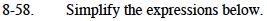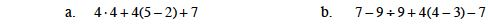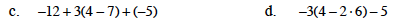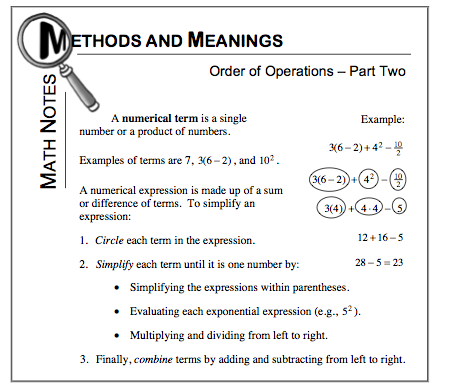### Home > MC1 > Chapter 8 > Lesson 8.2.3 > Problem8-58

8-58.For parts (a) through (d), refer to the Math Notes box below.Remember to calculate the value for each term before adding!

The answer to part (a) is 35.
Be sure to show your work!

The expression in parentheses should be simplified first.
Then remember to always multiply and divide before adding and subtracting.Try circling the terms before simplifying the expression.
This way you will be less likely to make a mistake!

Be careful adding and subtracting negative integers!

Make sure you simplify the expression in parentheses first!

19
Don't forget to keep track of those negative integers!